Time to Empty or Drain a Tank, Pond, Reservoir Containing Water or other Liquid

Compute time to empty (or lower) water or other liquid. Also can solve for discharge coefficient, orifice diameter (or area), tank diameter (or area), initial liquid depth, final liquid depth.

Register to enable "Calculate" button.

 Select orifice geometry: Circular Non-circular © 2014 LMNO Engineering, Select tank geometry: Circular Non-circular Research, and Software, Ltd. Select computation: Compute C Compute orifice d or a Compute tank D or A Compute initial depth, Hi Compute final depth, Hf Compute time Discharge Coefficient, C: www.LMNOeng.com Orifice Diameter, d: inch ft cm m Orifice Area, a: inch2 ft2 cm2 m2 Tank Diameter, D: inch ft cm m Tank Area, A: inch2 ft2 cm2 m2 Initial Depth, Hi: inch ft cm m Final Depth, Hf: inch ft cm m Time, t: sec min hour day

Units: cm=centimeter, ft=foot, hr=hour, m=meter, min=minute, sec=second.

Tank (or pond or reservoir) is open to the atmosphere. The tank can be cylindrical or other cross-section but must have the same cross-section for its entire height. The orifice can be circular or non-circular. If tank or orifice is non-circular, enter their area into our calculation. Hi , Hf , and h are measured vertically from the centerline of the orifice.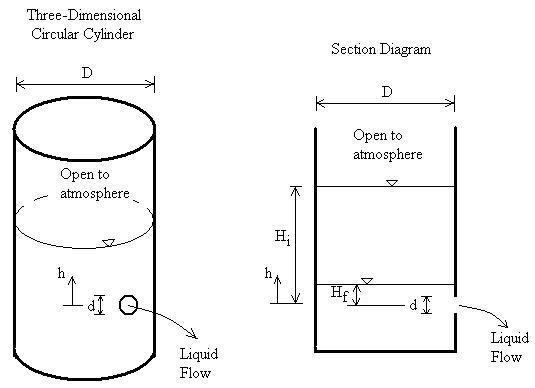Introduction
Water (or other liquid) draining out of a tank, reservoir, or pond is a common situation. Our calculation allows you to compute the time needed to lower the water from one depth to a lower depth or to empty the tank. Alternatively, the user can input a time and choose another variable to be computed - orifice coefficient, orifice diameter or orifice area, tank diameter or tank area, initial liquid depth, or final liquid depth.

Equations
If a tank and orifice are both at atmospheric pressure and the liquid is above the top of the orifice, the discharge (flow rate) out of the orifice is (Streeter et al., 1998, p. 467; Daugherty et al., 1985, p. 413):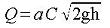Note: The above equation is valid if both the tank and orifice are at the same pressure, even if the pressure is not atmospheric

For a tank with a constant cross-sectional geometry A in the plan view (i.e. as you look down on it), substitute: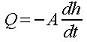Integrate h from Hi to Hf and integrate t from 0 to t, then solve for time t, which is the time required for the liquid to fall from Hi to Hf: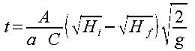If the tank is circular in plan view (i.e. looking down on it):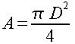If the orifice is circular: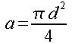Our calculation allows you to solve for any of the variables: C, d, a, D, A, Hi, Hf , or t. The orifice and tank can be either circular or non-circular. If non-circular, then the diameter dimension is not used in the calculation.

Validity
If h drops below the top of the orifice (for instance if Hf is below the top of the orifice), the method will give shorter emptying times than in reality. The program assumes that liquid is flowing out through the entire orifice area. However, when the liquid drops below the top of the orifice, flow is out of an area less than the full orifice area.

The tank and orifice discharge are assumed to be at the same pressure.

The tank is assumed to be of sufficiently larger area than the orifice so that friction effects from the tank wall are negligible; that is, A>10a as a rule of thumb suggested by LMNO Engineering.

Orifice Discharge Coefficient
Our steady state discharge from a tank page lists discharge coefficients for some orifice shapes (for water and similar liquids) from Dally et al. (1993), and they are repeated here. Similar values are shown in Daugherty et al. (1985, p. 415).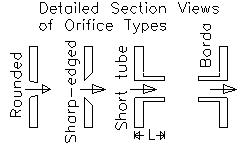Type:   Rounded     Sharp-edged       Short tube     Borda
C:          0.98                0.61                    0.8             0.51

The short tube value is valid for L ~ 2.5d. Borda is also known as "re-entrant" since it juts into the tank.

Variables
The units shown for the variables are SI (International System of Units); however, the equations above are valid for any consistent set of units. Our calculation allows a variety of units; all unit conversions are accomplished internally.
a = Orifice cross-sectional area (m2).
A = Tank cross-sectional area (m2).
C = Orifice discharge coefficient 0 < C < 1.0. C depends on the orifice geometry and in some cases the type of liquid.
d = Orifice diameter (m).
D = Tank diameter (looking down on it), (m).
g = Acceleration due to gravity, 9.8066 m/s2.
h = Vertical distance from centerline of orifice to liquid surface (m).
Hf = Final vertical distance from centerline of orifice to liquid surface (m).
Hi = Initial vertical distance from centerline of orifice to liquid surface (m).
Q = Discharge (flowrate) at any depth, h (m3/s).
t = Time for liquid in tank to change from a depth of Hi to a depth of Hf (seconds).

Error Messages given by calculation
"Need C > 0", "Need d > 0", "Need a > 0", "Need D > 0", "Need A > 0", "Need Hi > 0", "Need Hf ≥ 0", "Need Time > 0". These are initial checks. Discharge coefficient, orifice diameter (if circular), orifice area, tank diameter (if circular), initial liquid depth, and final liquid depth must be positive numbers.

"Need Hi > Hf". Initial depth must be larger than final depth.

"Best if A ≥ 10a". To ensure that tank friction is negligible, should have tank area much larger than orifice area.

"Need 0 < C ≤ 1". Discharge coefficient must be greater than 0.0 and less than or equal to 1.0. Typical value is 0.6 for water flowing through square-edged orifice.

"Infeasible input". This message can occur if the final depth is being computed and Hi0.5 < [t a C (g/2)0.5] / A

References
Dally, J. W., W. F. Riley, and K. G. McConnell. 1993. Instrumentation for Engineering Measurements. John Wiley and Sons, Inc. 2ed.

Daugherty, R. L., J. B. Franzini, and E. J. Finnemore. 1985. Fluid Mechanics with Engineering Applications. McGraw-Hill, Inc. 8ed.

Streeter, V. L., E. B. Wylie, and K. W. Bedford. 1998. Fluid Mechanics. McGraw-Hill, Inc. 9ed.

LMNO Engineering, Research, and Software, Ltd.
7860 Angel Ridge Rd.   Athens, Ohio 45701  USA   Phone: (740) 707-2614
LMNO@LMNOeng.com    http://www.LMNOeng.com

To:

Discharge from a tank (steady state)

Leak rate from pipe crack (liquids)

Bernoulli calculator

Orifice flow meter in pipe (D<5cm)

Orifice flow meter in pipe (D>5cm)

Unit Conversions Calculator

Other information:

Register